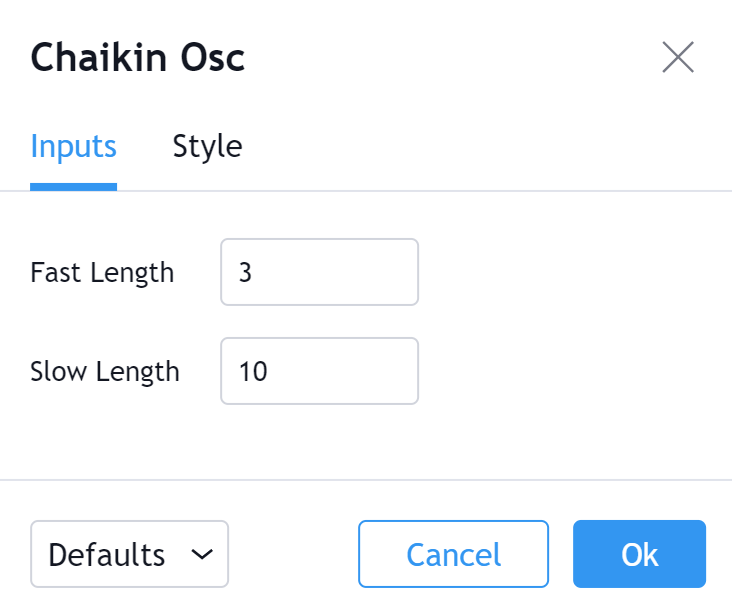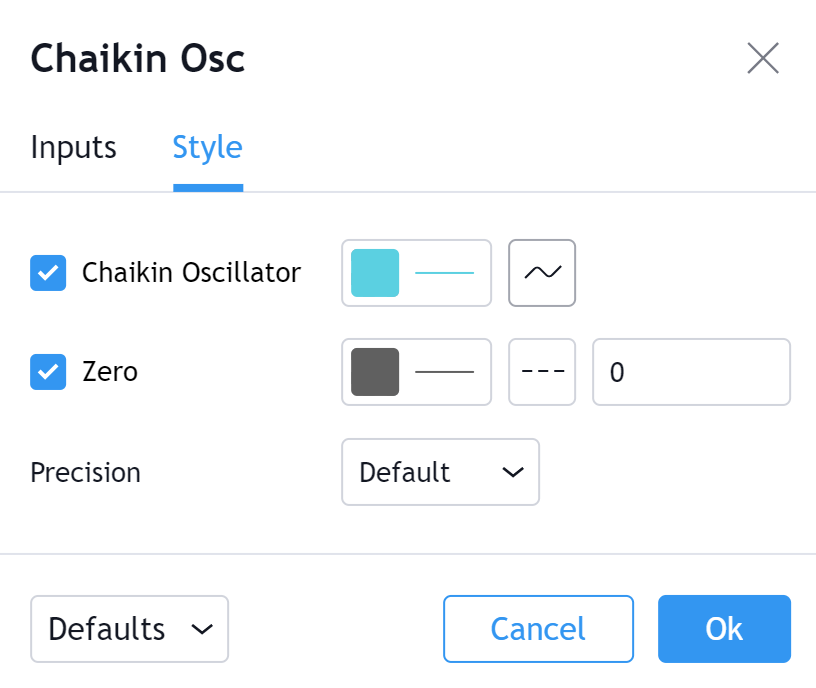# Chaikin Oscillator

#### Definition

The Chaikin Oscillator is, at its core, an indicator of an indicator. The Chaikin Oscillator takes Accumulation/Distribution (ADL) and applies two Exponential Moving Averages of varying length to the line. The Chaikin Oscillator's value is then derived by subtracting the longer term EMA of the ADL from the shorter term EMA of the ADL. Ultimately this serves as a way to measure the momentum of the ADL by plotting a line which fluctuates between positive and negative values. Being aware of changes in momentum can help a trader or technical analyst to anticipate trend changes since changes in momentum often precede changes in trend.

#### History

The Chaikin Oscillator technical indicator was created by famed stock analyst Marc Chaikin. The Chaikin Oscillator has become closely related to two of Chaikin’s other famous indicators; Chaikin Money Flow and Accumulation/Distribution (ADL).

#### Calculation

There are four steps in calculating The Chaikin Oscillator (this example is for a (3,10) Period):
1. Find the Money Flow Multiplier

`[(Close  -  Low) - (High - Close)] /(High - Low) = Money Flow Multiplier`

2. Calculate Money Flow Volume

`Money Flow Multiplier x Volume for the Period = Money Flow Volume`

`Previous ADL + Current Period Money Flow Volume = ADL`

4. Apply EMA (user defined periods) to the ADL to generate the Chaikin Oscillator

`(3-day EMA of ADL)  -  (10-day EMA of ADL) = Chaikin Oscillator`

#### The basics

Chaikin believed that buying and selling pressures could be determined by where a period closes in relation to its high/low range. If the period closes in the upper half of the range, then buying pressure is higher and if the period closes in the lower half of the range, then selling pressure is higher. This is what the Money Flow Multiplier determines (step 1 in the calculation above). The Money Flow Multiplier is the key to all of Chaikin's technical analysis tools. Money Flow Multiplier determines The Money Flow Volume, which in turn determines the direction of the ADL, which ultimately determines the direction and value of The Chaikin Oscillator.

As previously mentioned, The Chaikin Oscillator calculates a value that fluctuates between positive and negative values.

• When The Chaikin Oscillator's value is above 0, ADL's momentum and therefore buying pressure is higher.
• When The Chaikin Oscillator's value is below 0, ADL's momentum and therefore selling pressure is higher.

#### What to look for

##### Crosses

When The Chaikin Oscillator crosses the Zero Line, this can be an indication that there is an impending trend reversal.

Bullish Crosses occur when The Chaikin Oscillator crosses from Below the Zero Line to Above the Zero Line. Price then rises.

Bearish Crosses occur when The Chaikin Oscillator crosses from Above the Zero Line to Below the Zero Line. Price then falls.

##### Divergence

Chaikin Oscillator Divergence occurs when there is a difference between what price action is indicating and what The Chaikin Oscillator is indicating. These differences can be interpreted as an impending reversal.

Bullish Chaikin Oscillator Divergence is when price makes a new low but the Chaikin Oscillator makes a higher low.

Bearish Chaikin Oscillator Divergence is when price makes a new high but the Chaikin Oscillator makes a lower high.

#### Summary

Chaikin Oscillator is an indicator of an indicator. It uses Accumulation/Distribution (ADL) and takes it a step further. The ADL measure buying/selling pressure and the Chaikin Oscillator adds in the element of momentum. Because momentum often precedes changes in price or trend, it should always be taken into consideration by technical analysts when possible. However, like with most indicators, The Chaikin Oscillator is at its best when it is not used as a stand-alone technical indicator but in conjunction with additional indicators and chart analysis.

#### Inputs##### Fast Length

The time period to be used in calculating the shorter term EMA.

##### Slow Length

The time period to be used in calculating the longer term EMA.

#### Style##### Chaikin Oscillator

Can toggle the visibility of the Chaikin Oscillator Line as well as the visibility of a price line showing the actual current value of The Chaikin Oscillator. Can also select The Chaikin Oscillator Line's color, line thickness and visual type (Line is the default).

##### Zero

Can toggle the visibility of the Zero Line. Can also select the Zero Line's value, color, line thickness and visual type (Dashes are the default).

##### Precision

Sets the number of decimal places to be left on the indicator's value before rounding up. The higher this number, the more decimal points will be on the indicator's value.